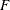# Introduction

### Vector Time Series Analysis

The VARMAX procedure enables you to model the dynamic relationship both between the dependent variables and between the dependent and independent variables. The VARMAX procedure includes the following features:

• several modeling features:

• vector autoregressive model

• vector autoregressive model with exogenous variables

• vector autoregressive and moving-average model

• Bayesian vector autoregressive model

• vector error correction model

• Bayesian vector error correction model

• GARCH-type multivariate conditional heteroscedasticity models

• criteria for automatically determining AR and MA orders:

• Akaike information criterion (AIC)

• corrected AIC (AICC)

• Hannan-Quinn (HQ) criterion

• final prediction error (FPE)

• Schwarz Bayesian criterion (SBC), also known as Bayesian information criterion (BIC)

• AR order identification aids:

• partial cross-correlations

• Yule-Walker estimates

• partial autoregressive coefficients

• partial canonical correlations

• testing the presence of unit roots and cointegration:

• Dickey-Fuller tests

• Johansen cointegration test for nonstationary vector processes of integrated order one

• Stock-Watson common trends test for the possibility of cointegration among nonstationary vector processes of integrated order one

• Johansen cointegration test for nonstationary vector processes of integrated order two

• model parameter estimation methods:

• least squares (LS)

• maximum likelihood (ML)

• model checks and residual analysis using the following tests:

• Durbin-Watson (DW) statistics

•test for autoregressive conditional heteroscedastic (ARCH) disturbance

•test for AR disturbance

• Jarque-Bera normality test

• Portmanteau test

• seasonal deterministic terms

• subset models

• multiple regression with distributed lags

• dead-start model that does not have present values of the exogenous variables

• Granger-causal relationships between two distinct groups of variables

• infinite order AR representation

• impulse response function (or infinite order MA representation)

• decomposition of the predicted error covariances

• roots of the characteristic functions for both the AR and MA parts to evaluate the proximity of the roots to the unit circle

• contemporaneous relationships among the components of the vector time series

• forecasts

• conditional covariances for GARCH models